Once the behavpy object is created, the print function  will just show your data structure. If you want to see your data and the metadata at once, use the built in method `.display()`

``````# first load your data and create a behavpy instance of it

df.display()``````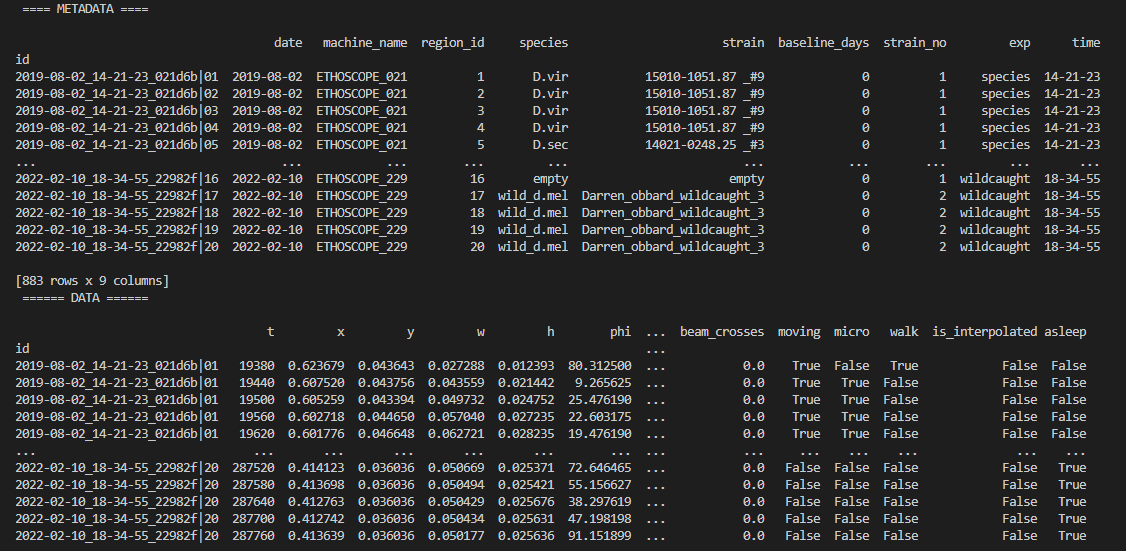You can also get quick summary statistics of your dataset with `.summary() `

``````df.summary()

# an example output of df.summary()
output:
behavpy table with:
individuals       675
metavariable         9
variables        13
measurements   3370075

# add the argument detailed = True to get information per fly
df.summary(detailed = True)

output:
data_points          time_range
id
2019-08-02_14-21-23_021d6b|01         5756   86400  ->  431940
2019-08-02_14-21-23_021d6b|02         5481   86400  ->  431940``````

Be careful with the pandas method `.groupby()`  as this will return a pandas object back and not a behavpy object. Most other common pandas actions will return a behavpy object.

Whilst summary statistics are good for a basic overview, visualising the variable of interest over time is usually a lot more informative.

##### Heatmaps

The first port of call when looking at time series data is to create a heatmap to see if there are any obvious irregularities in your experiments.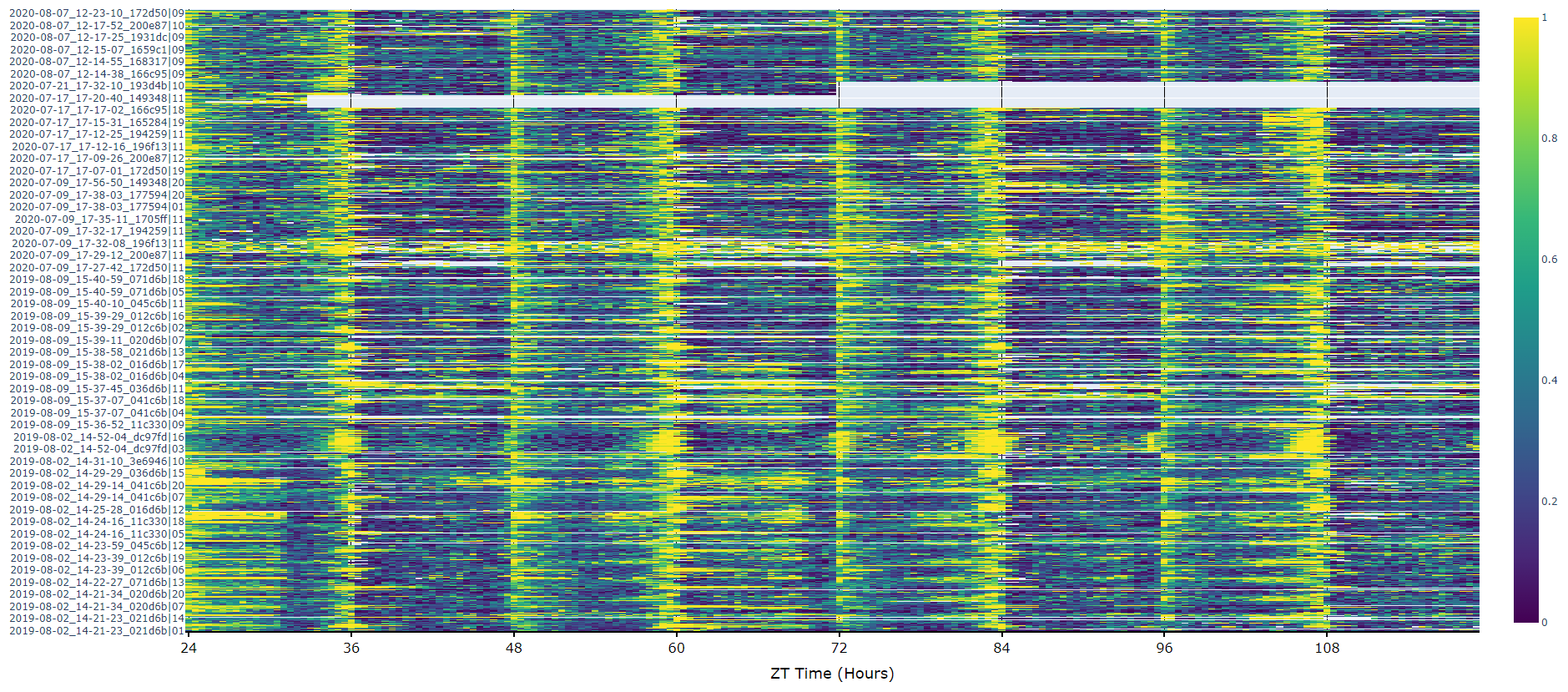##### Plots over time

For an aggregate view of your variable of interest over time, use the .plot_overtime() method to visualise the mean variable over your given time frame or split it into sub groups using the information in your metadata.

``````# If wrapped is True each specimens data will be aggregated to one 24 day before being aggregated as a whole. If you want to view each day seperately, keep wrapped False.
# A moving average is applied to the data with a window of 30 (30 mins if your time delta is 60 seconds). This can be changed with the parameter avg_window

df.plot_overtime(
variable = 'moving',
wrapped = True,
avg_window = 30
)

# the plots will show the mean with 95% confidence intervals in a lighter colour around the mean``````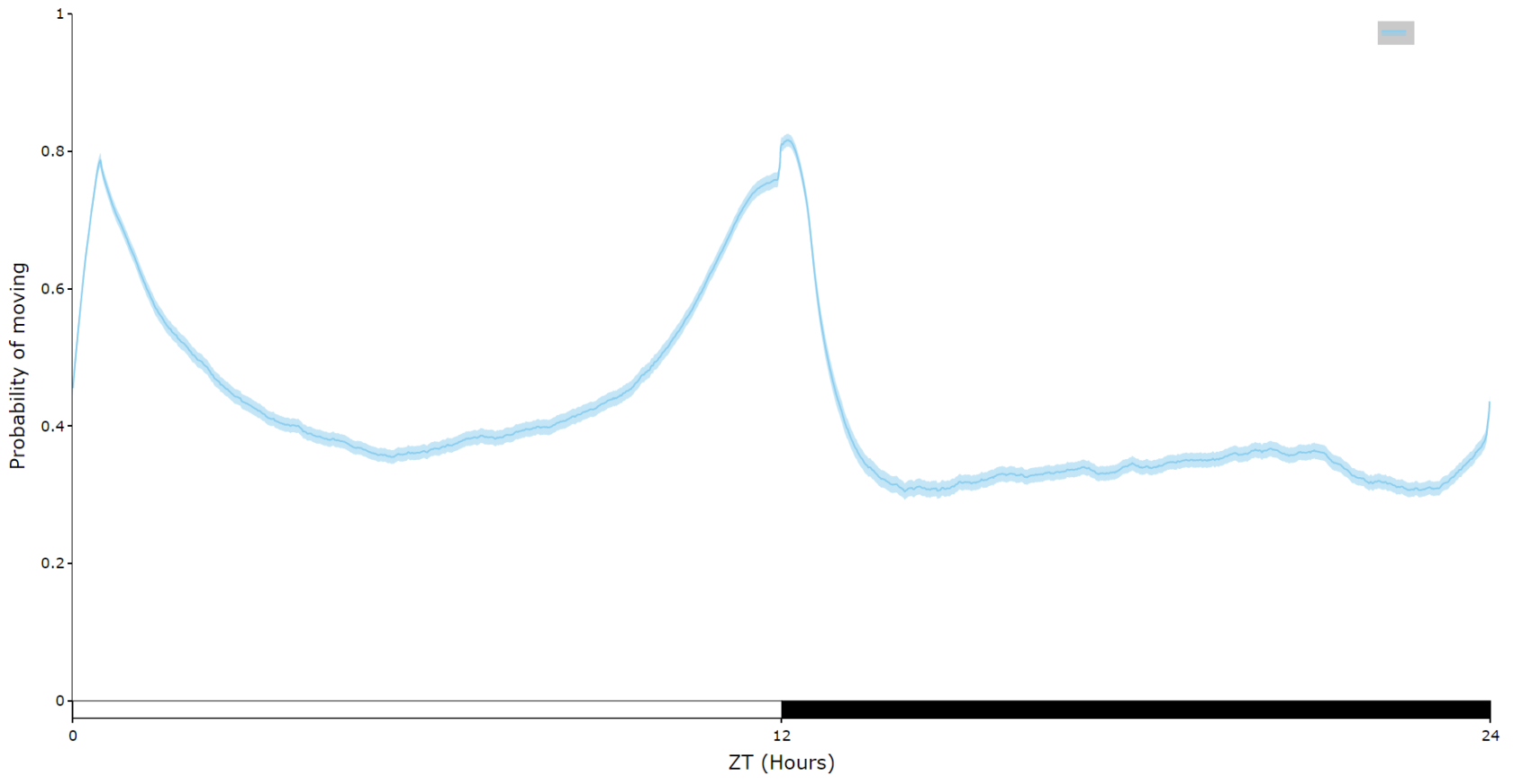``````# You can seperate out your plots by your specimen labels in the metadata. Specify which column you want fromn the metadata with facet_col and then specify which groups you want with facet_args
# What you enter for facet_args must be in a list and be exactly what is in that column in the metadata
# Don't like the label names in the column, rename the graphing labels with the facet_labels parameter. This can only be done if you have a same length list for facet_arg. Also make sure they are the same order

df.plot_overtime(
variable = 'moving',
facet_col = 'species',
facet_arg = ['D.vir', 'D.ere', 'D.wil', 'D.sec', 'D.yak'],
facet_labels = ['D.virilis', 'D.erecta', 'D.willistoni', 'D.sechellia', 'D.yakuba']
)

# if you're doing circadian experiments you can specify when night begins with the parameter circadian_night to change the phase bars at the bottom. E.g. circadian_night = 18 for lights off at ZT 18.``````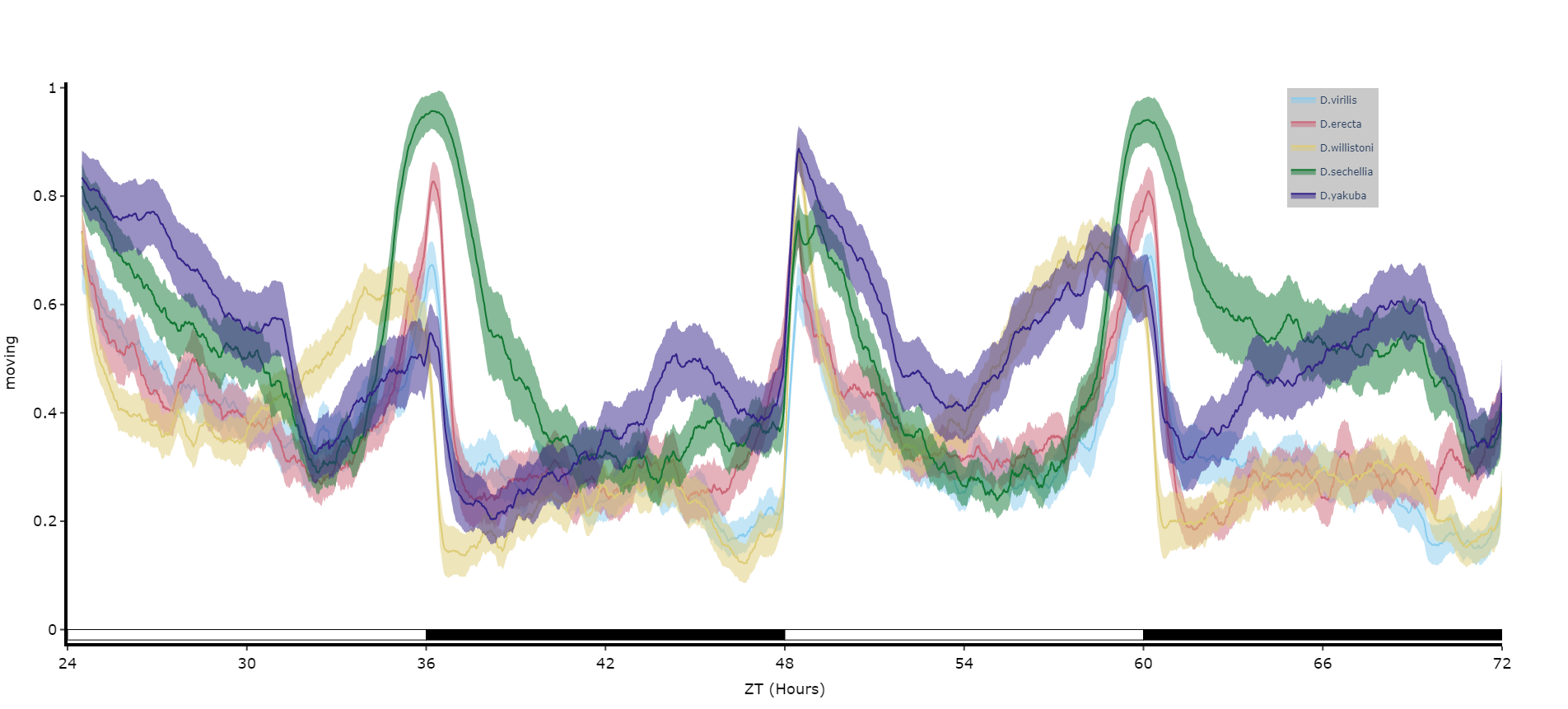##### Quantifying sleep

The plots above look nice, but we often want to quantify the differences to prove what our eyes are telling us. The method `.plot_quantify()` plots the mean and 95% CI of the specimens to give a strong visual indication of group differences.

``````# Quantify parameters are near identical to .plot_overtime()
# For all plotting methods in behavpy we can add grid lines to better view the data and you can also add a title, see below for how

df.plot_quantify(
variable = 'moving',
facet_col = 'species',
facet_arg = ['D.vir', 'D.ere', 'D.wil', 'D.sec', 'D.yak']
title = 'This is a plot for quantifying',
grids = True
)

# Tip! You can have a facet_col argument and nothing for facet_args and the method will automatically plot all groups within that column. Careful though as the order/colour might not be preserved in similar but different plots``````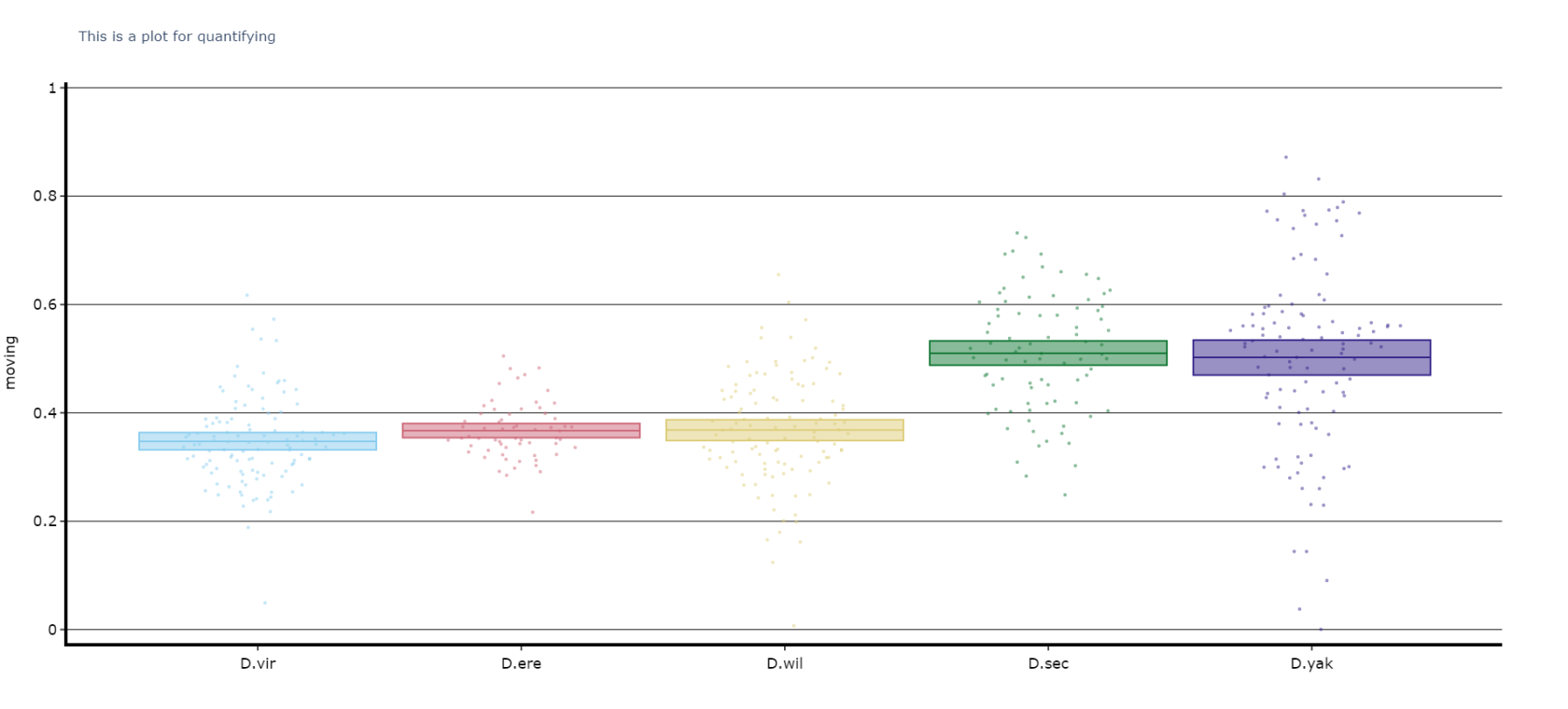If working in a python file workspace the plots should open automatically in your browser as a fully interactive plot. If in a Jupyter notebook it'll appear below the cell.
All plots are generated as plotly instances. You can save the plots as a `pdf` or `svg` for the best resolution or as html file to fully interact with them in the browser.
See a few pages down for examples on how to do this

##### Quantify day and night

Often you'll want to compare a variable between the day and night, particularly total sleep. This variation of `.plot_quantify()` will plot the mean and 96% CI of a variable for a user defined day and night.

``````# Quantify parameters are near identical to .plot_quantify() with the addtion of day_length and lights_off which takes (in hours) how long the day is for the specimen (defaults 24) and at what point within that day the lights turn off (night, defaults 12)

df.plot_day_night(
variable = 'asleep',
facet_col = 'species',
facet_arg = ['D.vir', 'D.ere', 'D.wil', 'D.sec', 'D.yak'],
facet_labels = ['D.virilis', 'D.erecta', 'D.willistoni', 'D.sechellia', 'D.yakuba'],
day_length = 24,
lights_off = 12
)``````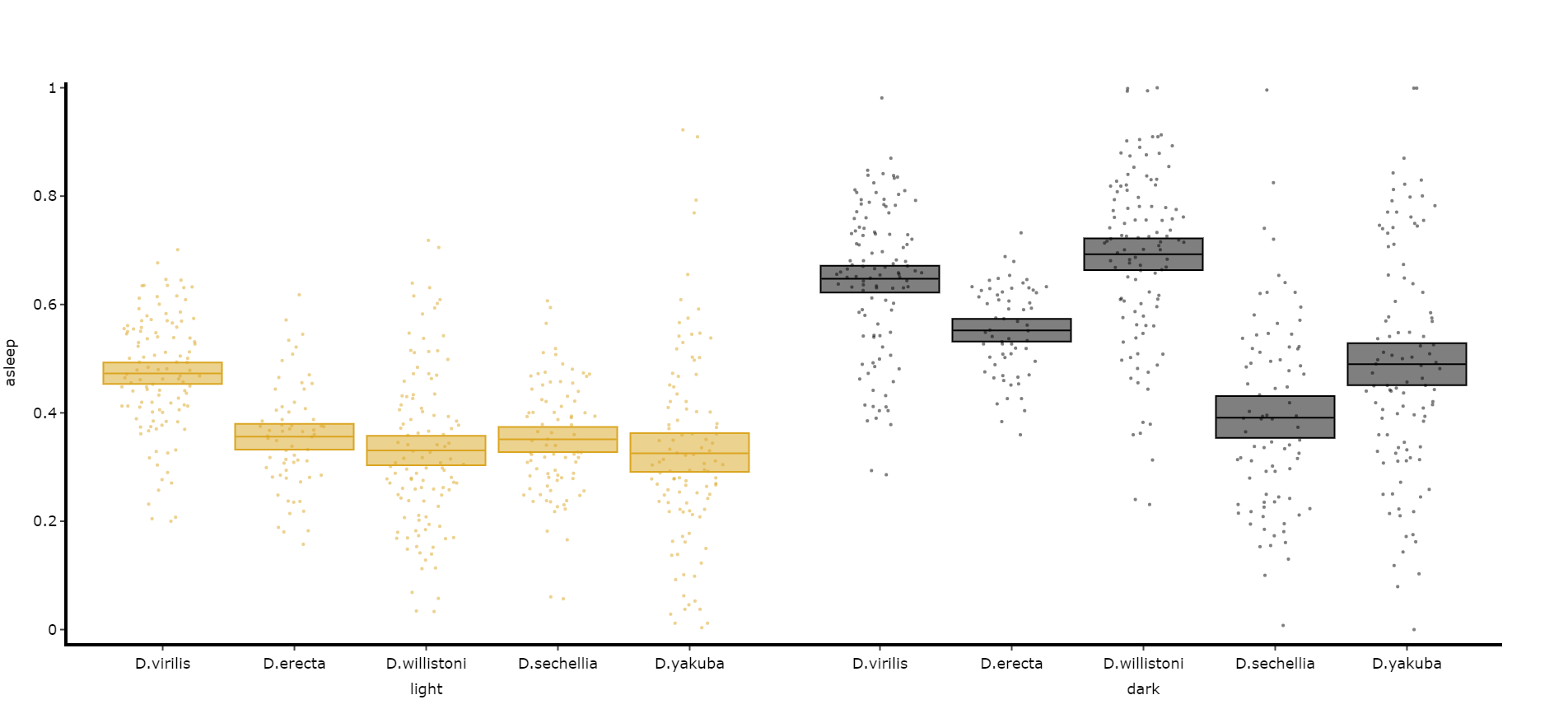##### Compare variables

You may sometimes want to compare different but similar variables from your experiment, often comparing them across different experimental groups. Use `.plot_compare_variables()` to compare up  to 24 different variables (we run out of colours after that...).

``````# Like the others it takes the regualar arguments. However, rather than the variable parameter it's variables, which takes a list of strings of the different columns you want to compare. The final variable in the list will have it's own y-axis on the right-hand side, so save this one for different scales.

# The most often use of this with the ethoscope data is to compare micro movements to walking movements to better understand the locomotion of the specimen, so lets look at that.

df.plot_day_night(
variables = ['mirco', 'walk']
facet_col = 'species',
facet_arg = ['D.vir', 'D.ere', 'D.wil', 'D.sec', 'D.yak'],
facet_labels = ['D.virilis', 'D.erecta', 'D.willistoni', 'D.sechellia', 'D.yakuba']
)``````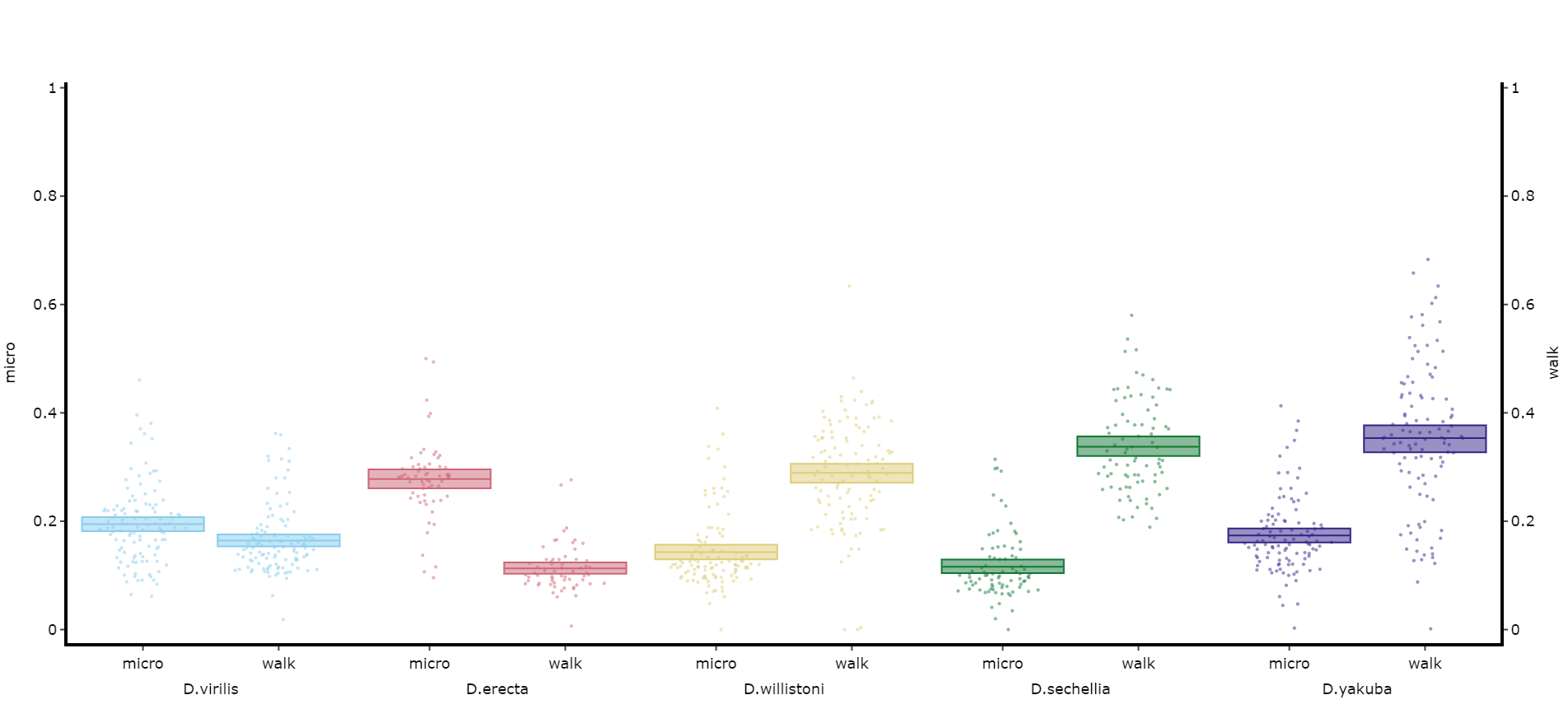``````# Lets add the velocity of the specimen to see how it looks with different scales.

df.plot_day_night(
variables = ['mirco', 'walk', 'mean_velocity']
facet_col = 'species',
facet_arg = ['D.vir', 'D.ere', 'D.wil', 'D.sec', 'D.yak', 'D.sims'],
facet_labels = ['D.virilis', 'D.erecta', 'D.willistoni', 'D.sechellia', 'D.yakuba', 'D.Simulans'],
# Add grids to the plot so we can better see how the plots align
grids = True
)
``````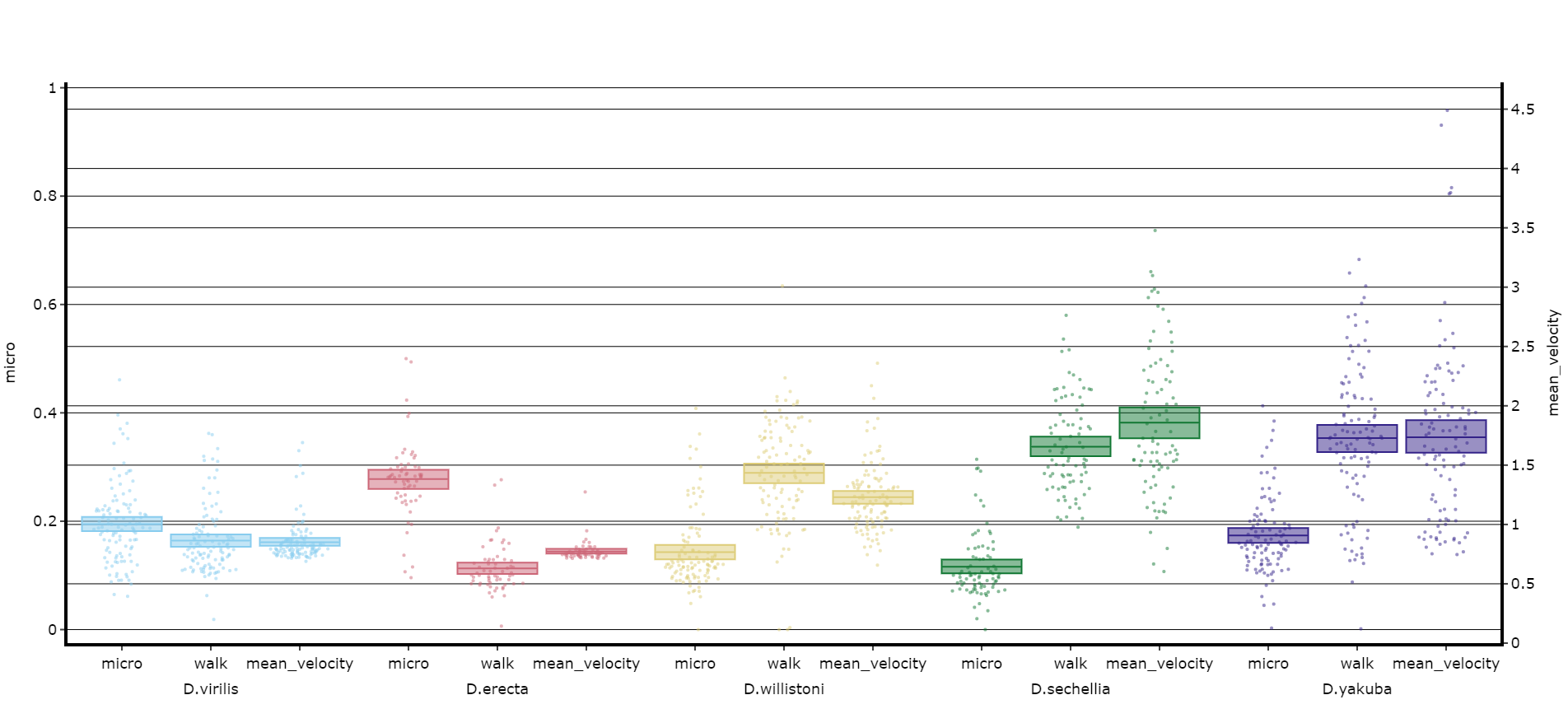##### Saving plots

When saving your plots it's recommended to save if as a `pdf` if you want to use it in documents. This will create a static image, but retain the structure. However, sometimes you'll want to save it as a html file, as this can be loaded in your browser as a fully interactive graph, the biggest strength of plotly! This is most useful in jupyter notebooks as the plot below the cell can be quite small.

``````# to save a file just provide the path and file name of the plot, change the ending to whatever you want the file to be e.g. .pdf, .html, .png

df.plot_overtime(
variable = 'moving',
facet_col = 'species',
facet_arg = ['D.vir', 'D.ere', 'D.wil', 'D.sec', 'D.yak'],
facet_labels = ['D.virilis', 'D.erecta', 'D.willistoni', 'D.sechellia', 'D.yakuba'],
save = '/home/user/folder/plot.pdf'
)``````